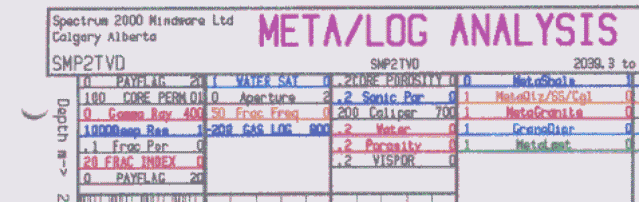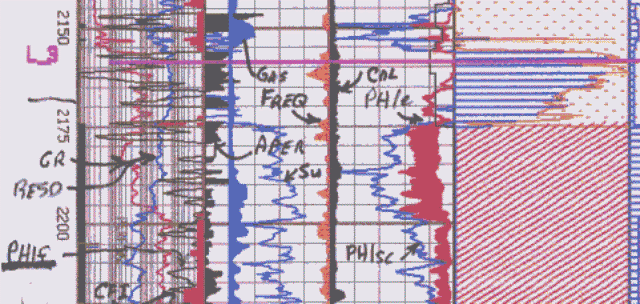Calculating Fracture Intensity - Crain’s Method
The various log derived fracture indicators can be merged through a computer program which allows a wide variety of inputs. The input curves are assigned a threshold value, a median value, and a maximum probability as a fracture detector. In addition, each input is weighted according to its correlation to natural fracturing in the specific area. This form of program lends itself to a small rule based expert system.

In most areas, the major weighting is assigned to the shear attenuation from the sonic waveforms and to dipmeter differential conductivity. Lesser weighting is assigned to compressional attenuation, caliper rugosity, density correction, deep to shallow resistivity ratio, and uranium content. Where oblique fracturing are expected, the shear input may be weighted lower and the compressional input weighted higher. The output of the program is a fracture probability curve.

A simple form of the equation would look something like this

1. CFI = ((RESS<RESD) + (PHID>PHIN+0.05) + (DTC>200) + (GR>150) + (PE>5.5) + (CAL>250)
+ (DCOR>250) + DELTA_CAL>50)) / NTEST

Where:
CFI = calculated fracture index (fractional)
RESS = shallow resistivity
RESD = deep resistivity
PHID = density porosity
PHIN = neutron porosity
DTC= sonic travel time
GR = gamma ray
PE = photo electric effect
CAL = caliper
DCOR = density correction
DELTA_CAL = differential caliper
NTEST = number of thresholds tested

This equation is written for a specific case; curves can be added or deleted and thresholds adjusted to suit the circumstances.

In the above sample, units are Metric – CAL in mm, DTC = usec/m, and DCOR in kg/m3.

The equation is trying to quantify the fracture location rules shown in the examples of how each log curve responds to fractures.

You need to look at the logs to see what they are doing – are RESS spikes lower or higher than RESD? Do all resistivity curves spike to lower values? Set CAL, DCOR, and DELT trigger levels apptopriately for the log response and log scales.

Whether RESS is higher or lower than RESD will depend on mud resistivity and zone is wey or oil/gas. If zone is wet, fresh mud gives RESS > RESD as the mud invades the fracture. Fresg mud in fractures in oil zone – usually RESS < RESD. Salt mud and oil-base muds would behave differentlt.

In the example shown above, all curves have equal weight, and the amount of excursion of a curve beyond its threshold is not considered. The result is normalized between 0.0 and 1.0 by the value of NTEST. More elaborate fracture intensity indicators are common, using the amount of the curve excursion above the thresld to weight that vurve's contribution to the total fracture intensity.Open hole fracture indicator (CFI) compared to FMI results

The CFI curve and micro-scanner fracture intensity (frequency or fractures per meter, labeled FREQ) track each other reasonable well. There is a strong correlation between the FMI data, which represents ‘ground truth’, and the CFI curve. This does not always happen and the CFI must be calibrated to each specific case.

Note the Fracture Aperture (APER) and Fracture Porosity (PHIf) curve values are very small, but typical of most fractured reservoirs. Matrix porosity (PHIe) is significant and overwhelms the fracture pore volume.

It is sometimes possible to relate the sum of CFI over an interval to drill stem test flow capacity (KH) or to well productivity (IPR or AOF). CFI can also be compared and calibrated to fracture intensity (fractures per meter) from formation micro-scanner processing. This is useful when only a few wells have FMS or FMI data while others do not.

Page Views ---- Since 01 Jan 2015
Copyright 2023 by Accessible Petrophysics Ltd.
CPH Logo, "CPH", "CPH Gold Member", "CPH Platinum Member", "Crain's Rules", "Meta/Log", "Computer-Ready-Math", "Petro/Fusion Scripts" are Trademarks of the Author